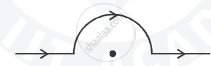Advertisement Remove all ads

# A straight wire carrying a current of 5 A is bent into a semicircular arc of radius 2 cm as shown in the  figure. Find the magnitude and direction of the magnetic field at the centre of the arc. - Physics

Answer in Brief

A straight wire carrying a current of 5 A is bent into a semicircular arc of radius 2 cm as shown in the  figure. Find the magnitude and direction of the magnetic field at the centre of the arc.Advertisement Remove all ads

#### Solution

Current I = 5A, radius r = 2 cm = 2 x 10-2 m, Number of turns = N = 1/2
So, magnetic field due to semi-circular arc is

b = (mu_0 NI)/(2r) = (4 pi xx 10^(-7) xx 0.5 xx5)/(2 xx 2 xx 10^(-2)) T = 7.85 xx 10^(-5) T

The magnetic field due to the straight part of the wire is zero at the centre as its line intersects the centre.
Hence net magnetic field =  7.85×10-5
The direction of the this magnetic field is perpendicular to the plane of the paper and directed inside .

Is there an error in this question or solution?
Advertisement Remove all ads

#### APPEARS IN

Advertisement Remove all ads

#### Video TutorialsVIEW ALL 

Advertisement Remove all ads
Share
Notifications

View all notifications

Forgot password?